Anzeige

# Class 3.pdf

10. Aug 2022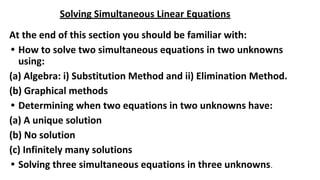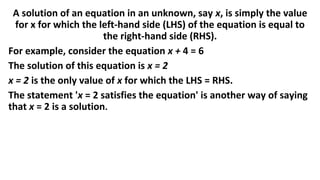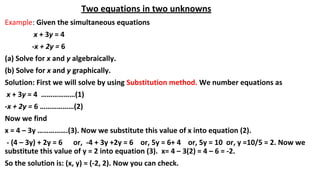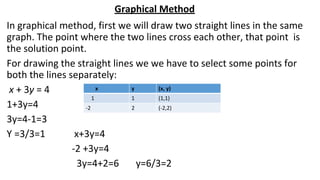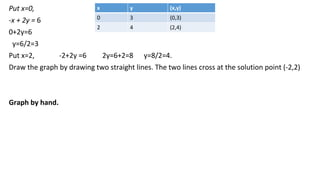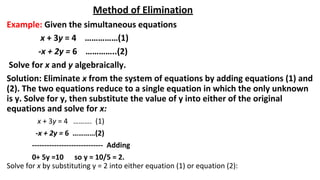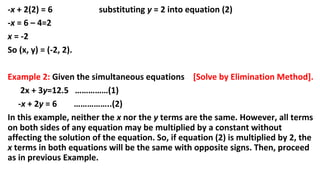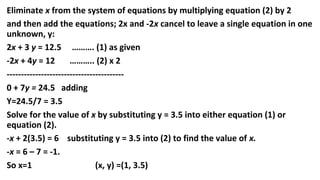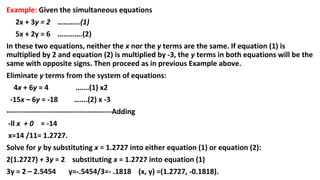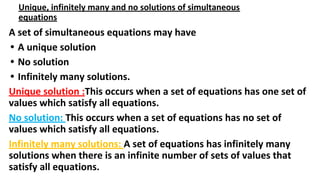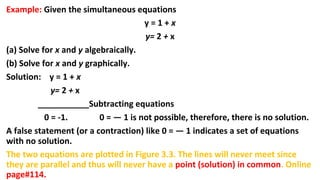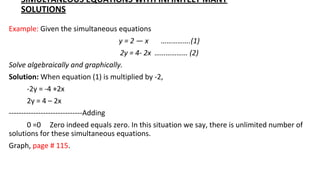1 von 12
Anzeige

### Class 3.pdf

1. Solving Simultaneous Linear Equations At the end of this section you should be familiar with: • How to solve two simultaneous equations in two unknowns using: (a) Algebra: i) Substitution Method and ii) Elimination Method. (b) Graphical methods • Determining when two equations in two unknowns have: (a) A unique solution (b) No solution (c) Infinitely many solutions • Solving three simultaneous equations in three unknowns.
2. A solution of an equation in an unknown, say x, is simply the value for x for which the left-hand side (LHS) of the equation is equal to the right-hand side (RHS). For example, consider the equation x + 4 = 6 The solution of this equation is x = 2 x = 2 is the only value of x for which the LHS = RHS. The statement 'x = 2 satisfies the equation' is another way of saying that x = 2 is a solution.
3. Two equations in two unknowns Example: Given the simultaneous equations x + 3y = 4 -x + 2y = 6 (a) Solve for x and y algebraically. (b) Solve for x and y graphically. Solution: First we will solve by using Substitution method. We number equations as x + 3y = 4 ………………(1) -x + 2y = 6 ………………(2) Now we find x = 4 – 3y …………….(3). Now we substitute this value of x into equation (2). - (4 – 3y) + 2y = 6 or, -4 + 3y +2y = 6 or, 5y = 6+ 4 or, 5y = 10 or, y =10/5 = 2. Now we substitute this value of y = 2 into equation (3). x= 4 – 3(2) = 4 – 6 = -2. So the solution is: (x, y) = (-2, 2). Now you can check.
4. Graphical Method In graphical method, first we will draw two straight lines in the same graph. The point where the two lines cross each other, that point is the solution point. For drawing the straight lines we we have to select some points for both the lines separately: x + 3y = 4 1+3y=4 3y=4-1=3 Y =3/3=1 x+3y=4 -2 +3y=4 3y=4+2=6 y=6/3=2 x y (x, y) 1 1 (1,1) -2 2 (-2,2)
5. Put x=0, -x + 2y = 6 0+2y=6 y=6/2=3 Put x=2, -2+2y =6 2y=6+2=8 y=8/2=4. Draw the graph by drawing two straight lines. The two lines cross at the solution point (-2,2) Graph by hand. x y (x,y) 0 3 (0,3) 2 4 (2,4)
6. Method of Elimination Example: Given the simultaneous equations x + 3y = 4 ……………(1) -x + 2y = 6 …………..(2) Solve for x and y algebraically. Solution: Eliminate x from the system of equations by adding equations (1) and (2). The two equations reduce to a single equation in which the only unknown is y. Solve for y, then substitute the value of y into either of the original equations and solve for x: x + 3y = 4 ………. (1) -x + 2y = 6 …………(2) ----------------------------- Adding 0+ 5y =10 so y = 10/5 = 2. Solve for x by substituting y = 2 into either equation (1) or equation (2):
7. -x + 2(2) = 6 substituting y = 2 into equation (2) -x = 6 – 4=2 x = -2 So (x, y) = (-2, 2). Example 2: Given the simultaneous equations [Solve by Elimination Method]. 2x + 3y=12.5 ……………(1) -x + 2y = 6 ……………..(2) In this example, neither the x nor the y terms are the same. However, all terms on both sides of any equation may be multiplied by a constant without affecting the solution of the equation. So, if equation (2) is multiplied by 2, the x terms in both equations will be the same with opposite signs. Then, proceed as in previous Example.
8. Eliminate x from the system of equations by multiplying equation (2) by 2 and then add the equations; 2x and -2x cancel to leave a single equation in one unknown, y: 2x + 3 y = 12.5 ………. (1) as given -2x + 4y = 12 ……….. (2) x 2 ----------------------------------------- 0 + 7y = 24.5 adding Y=24.5/7 = 3.5 Solve for the value of x by substituting y = 3.5 into either equation (1) or equation (2). -x + 2(3.5) = 6 substituting y = 3.5 into (2) to find the value of x. -x = 6 – 7 = -1. So x=1 (x, y) =(1, 3.5)
9. Example: Given the simultaneous equations 2x + 3y = 2 …………(1) 5x + 2y = 6 ………….(2) In these two equations, neither the x nor the y terms are the same. If equation (1) is multiplied by 2 and equation (2) is multiplied by -3, the y terms in both equations will be the same with opposite signs. Then proceed as in previous Example above. Eliminate y terms from the system of equations: 4x + 6y = 4 …….(1) x2 -15x – 6y = -18 …….(2) x -3 -------------------------------------------Adding -ll x + 0 = -14 x=14 /11= 1.2727. Solve for y by substituting x = 1.2727 into either equation (1) or equation (2): 2(1.2727) + 3y = 2 substituting x = 1.2727 into equation (1) 3y = 2 – 2.5454 y=-.5454/3=- .1818 (x, y) =(1.2727, -0.1818).
10. Unique, infinitely many and no solutions of simultaneous equations A set of simultaneous equations may have • A unique solution • No solution • Infinitely many solutions. Unique solution :This occurs when a set of equations has one set of values which satisfy all equations. No solution: This occurs when a set of equations has no set of values which satisfy all equations. Infinitely many solutions: A set of equations has infinitely many solutions when there is an infinite number of sets of values that satisfy all equations.
11. Example: Given the simultaneous equations y = 1 + x y= 2 + x (a) Solve for x and y algebraically. (b) Solve for x and y graphically. Solution: y = 1 + x y= 2 + x ___________Subtracting equations 0 = -1. 0 = — 1 is not possible, therefore, there is no solution. A false statement (or a contraction) like 0 = — 1 indicates a set of equations with no solution. The two equations are plotted in Figure 3.3. The lines will never meet since they are parallel and thus will never have a point (solution) in common. Online page#114.
12. SIMULTANEOUS EQUATIONS WITH INFINITELY MANY SOLUTIONS Example: Given the simultaneous equations y = 2 — x …………….(1) 2y = 4- 2x ……………… (2) Solve algebraically and graphically. Solution: When equation (1) is multiplied by -2, -2y = -4 +2x 2y = 4 – 2x ------------------------------Adding 0 =0 Zero indeed equals zero. In this situation we say, there is unlimited number of solutions for these simultaneous equations. Graph, page # 115.
Anzeige